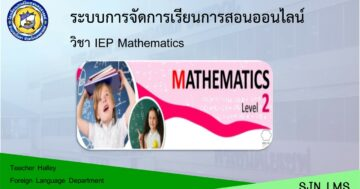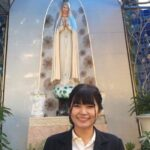# Mathematics IEP P.2 2nd Semester## เกี่ยวกับคอร์ส

Course Description
Department of Mathematics 2                                                 Intensive Science and Technology 2
Time 40 Hours                                                                             Primary 2
Students are able to tell the numbers of things, represent things in determined numbers,
read and write Hindu-Arabic numbers, Thai numbers, and word form of numbers not exceeding
1,000 and 0; count the numbers by 2s, 5s, 10s and 100s; differentiate even and odd numbers;
tell place value of the number in each digit; write expanded form of the number; compare and
arrange the numbers; find the sum and the difference not exceeding 1,000; understand the
relationship between addition and subtraction; find the value of the variable in the number
create the word problems; understand the meaning of multiplication and subtraction; find the
product in the multiplication sentences of one-place and one or two-place numbers; understand
relationship between multiplication and division; find the 1-digit quotient with the 1
the variables in the multiplication and division sentences; solve the word pro
multiplication and division; find the result in the combination sentences and word problems of
addition, subtraction, multiplication and division; tell the number patterns that increase or decrease
by 2s, 5s and 100s; categorize the properties of polygons, circles, and ovals; draw the two-
dimensional geometric shapes by using geometric forms; measure the length in meters and
centimeters; estimate the length in the unit of meters; compare the length using the relationship
between the units of meters and centimeters; solve the word problems about length with the units
of meters and centimeters; tell time in o’clock and minutes; tell and compare period of time in
hours and minutes; read the calendar; solve the word problems about time; measure weight in
kilograms/grams and kilograms/kheeds; estimate the weight in the unit of kilograms; compare
weight using the relationship between the units of kilograms/grams and kilograms/kheeds; solve
the word problems about weight with the units of kilograms/grams and kilograms/kheeds; measure
the volume and capacity with non-standard and standard units of teaspoons, tablespoons, cups
and liters; compare and solve the word problem about the volume and capacity with the units of
teaspoons, tablespoons, cups and liters; read and use the data from the pictograph to solve the
word problems.

### What Will You Learn?

• students will be able to understand the diversity of presenting numbers, system of numbers, the operations of numbers, the properties of the operations and their application00:00

00:00
00:00

00:00
00:00
00:00

00:00

00:00
00:00

### Student Ratings & ReviewsNo Review Yet